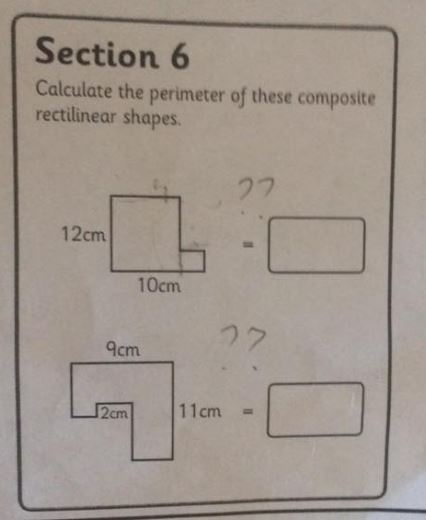## Geometry Tutorial

#### Intro

Seemingly complex, but actually rather simple problem involving perimeter

#### Sample Problem

Question source#### SolutionThe first one can be calculated as follows, a+b+c+d+e+f = a+(b+c)+(d+e)+f = a+f+a+f
= 12+10+12+10 = 44, where you can see that the sum of sides b and c is equivalent to side f, and the sum of sides d and e is equivalent to side a

The second one can be calculated in a similar manner. Here it gets a little complicated though, as the shape overlaps itself a little bit. The 2cm corresponds to the lengths highlighted in red which are overlapping bits in the regular rectangle

a+b+c+d+e+f+g = a+b+(c+d+e)+(f+g)+2+2 = a+b+a+b+2+2 = 9+11+9+11+2+2 = 44.